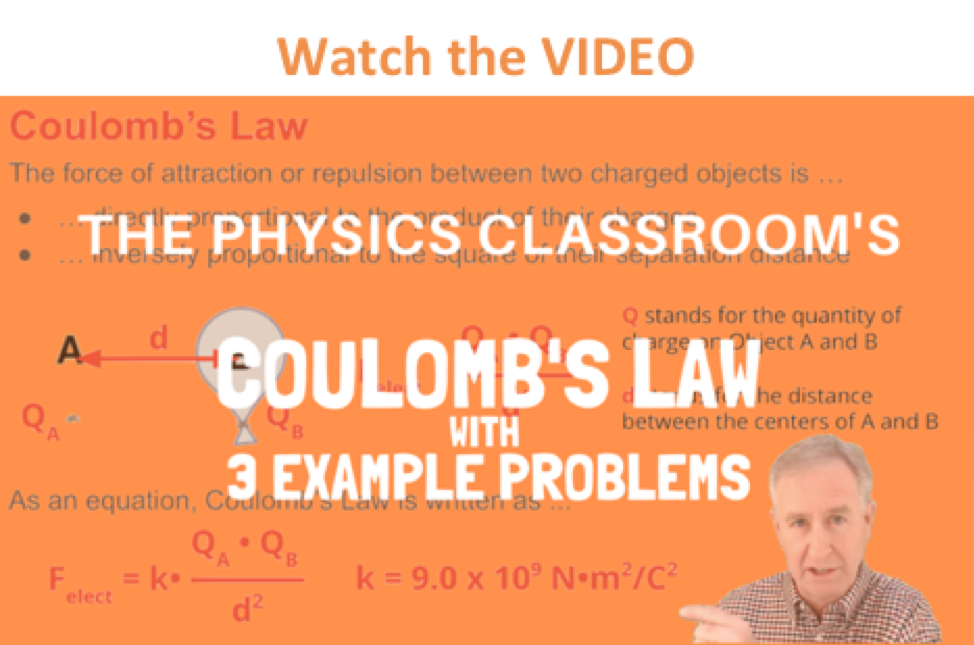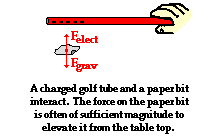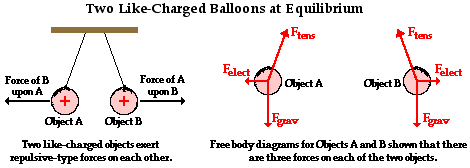Static Electricity - Lesson 3 - Electric Force

# Charge Interactions RevisitedPreviously in Lesson 1, the interactions between charged objects of like charge and opposite charge were discussed. At that time, the two fundamental charge interactions were stated: oppositely charged objects attract and like charged objects repel. These mutual interactions resulted in an electric force between the two charged objects. This force is commonly observed in physics lab activities and classroom demonstrations. In Lesson 3, we will explore these charge interactions in more detail and begin to quantify it. We will introduce Coulomb's law of electric force, which is a mathematical expression of these charge interactions. Ultimately, we will combine Coulomb's law of electric force with Newton's laws of motion to attempt to explain the variety of charge interactions that we have observed throughout the unit.

### Charge Interactions are Forces

It is possible that you might have watched two balloons repel each other a dozen or more times and never even thought of the balloon interaction as being a force. Or perhaps you have used a plastic golf tube or other object to raise small paper bits off the lab table and never thought of Newton's laws of motion. Perhaps even now you're thinking, "Why should I? That was the Newton's Laws unit and this is the Static Electricity unit." True. However, the physical world that we study does not separate itself into separate topics, as we teachers and students are prone to do. Physics has an amazing way of fitting together in a seamless fashion. The information that you have forgotten about from the Newton's laws unit has a mischievous way of creeping up on you in other units. That forgetfulness (or negligence or mere ignorance) will haunt you as you try to learn new physics. The more physics that you learn (as in really learn), the more that you come to recognize that the pieces of the physics puzzle fit together to form a unified picture of the world of sight, sound, touch and feel. Here we will explore how Newton's laws of motion fit together with the interaction of charged objects.

Suppose that you hold a charged plastic golf tube above a handful of paper bits at rest on the table. The presence of the charged tube is likely to polarize a few bits of paper and then begin to exert an upward pull upon them. The attraction between a charged tube and a polarized (yet neutral) paper bit is an electrical force - Felect. Like all the forces studied in The Physics Classroom, the electrical force is apush or pull exerted upon an object as a result of an interaction with another object. The interaction is the result of electrical charges and thus it is called an electrical force.

Unlike many forces that we study, the electrical force is a non-contact force - it exists despite the fact that the interacting objects are not in physical contact with each other. The two objects can act over a separation distance and exert an influence upon each other. In this case, the plastic golf tube pulls upward upon the paper bit and a paper bit pulls downward upon the golf tube. In this case, the force is significantly small. If you were holding the golf tube, you would not likely sense the downward pull exerted upon it by the paper bit. On the other hand, the force is often large enough to either balance or even overwhelm the downward pull of gravity (Fgrav) upon the paper bit and cause it to be elevated or even accelerated off the table. Of course the actual result of the force upon the paper bit is related to Newton's laws and a free-body analysis. If at any moment, the electrical force were greater in magnitude than the gravitational force, the paper bit would be accelerated upward. And if at any moment, the electrical force is equal in magnitude to the gravitational force, the paper bit will be suspended (or levitated) in midair. The paper bit would be said to be at equilibrium.

Now consider the case of the rubber balloons hanging by light threads from the ceiling. If each balloon is rubbed in the same manner (with animal fur), they each become negatively charged and exert a repulsive effect upon each other. This charge interaction results in a force upon each balloon that is directed away from the balloon with which it interacts. Once more, we can identify this repulsive effect as an electrical force. This electrical force joins two other forces that act upon the balloon - the tension force and a force of gravity. Since the balloons are at rest, the three forces must balance each other such that the net force is zero. A more in-depth analysis of this force cancellation requires a discussion of vectors and is saved for the end of Lesson 3.Both of these examples illustrate how the interaction between two charges results in a mutual force acting upon the charged objects. An electrical interaction is a force which, like any force, can be analyzed using a free-body diagram and Newton's laws. But what factors affect the magnitude of this force? Is there an equation that can be used to quantify it the same manner as was done for the force of gravity (Fgrav = m•g) and the force of friction (Ffrict = mu•Fnorm)? The answer is Yes! Coulomb's law holds the key to understanding the answer to these questions. It is the topic of the next section of Lesson 3.

### We Would Like to Suggest ...Sometimes it isn't enough to just read about it. You have to interact with it! And that's exactly what you do when you use one of The Physics Classroom's Interactives. We would like to suggest that you combine the reading of this page with the use of our Coulomb's Law Interactive an/or our Put the Charge in the Goal Interactive. Both Interactives can be found in the Physics Interactives section of our website. These Interactives provide a means of interactively exploring action-at-a-distance in an engaging environment.

Next Section:
Jump To Next Lesson:

Follow Us